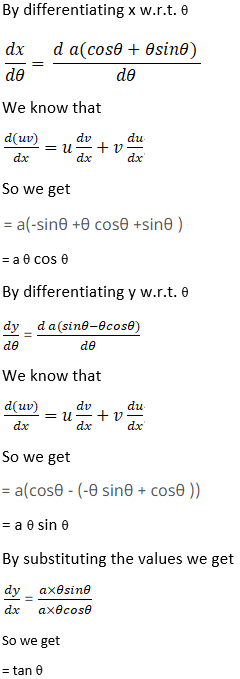# RS Aggarwal Solutions for Class 12 Maths Chapter 10: Differentiation Exercise 10I

The main topic which is discussed under this exercise is derivatives of parametric functions along with solved examples. The solutions for the exercise wise problems are prepared by experts at BYJU’S to help students perform well in the board exam. The problems are solved in a stepwise manner according to the exam pattern. Students can obtain RS Aggarwal Solutions Class 12 Maths Chapter 10 Differentiation Exercise 10I free PDF, from the links which are given here.

## RS Aggarwal Solutions for Class 12 Maths Chapter 10: Differentiation Exercise 10I Download PDF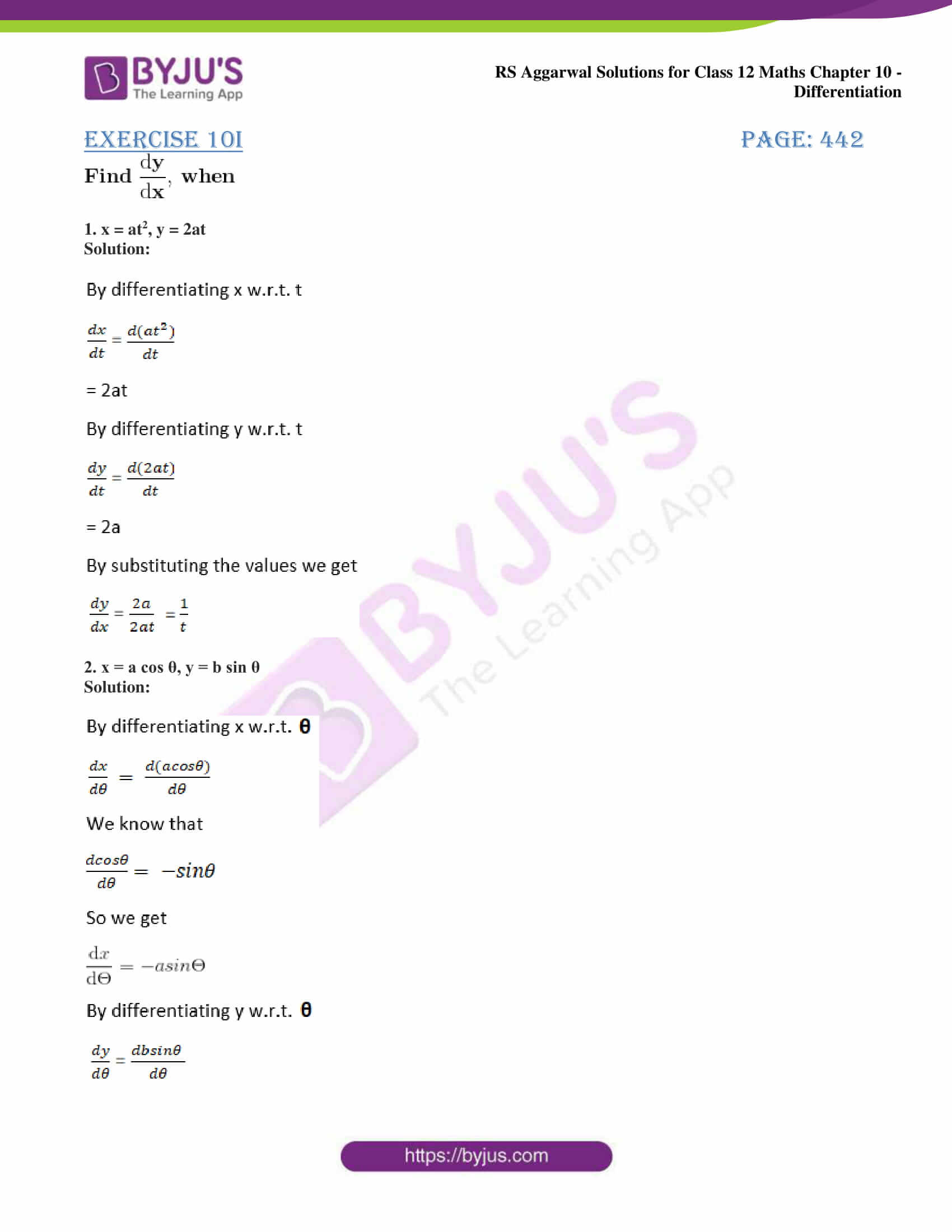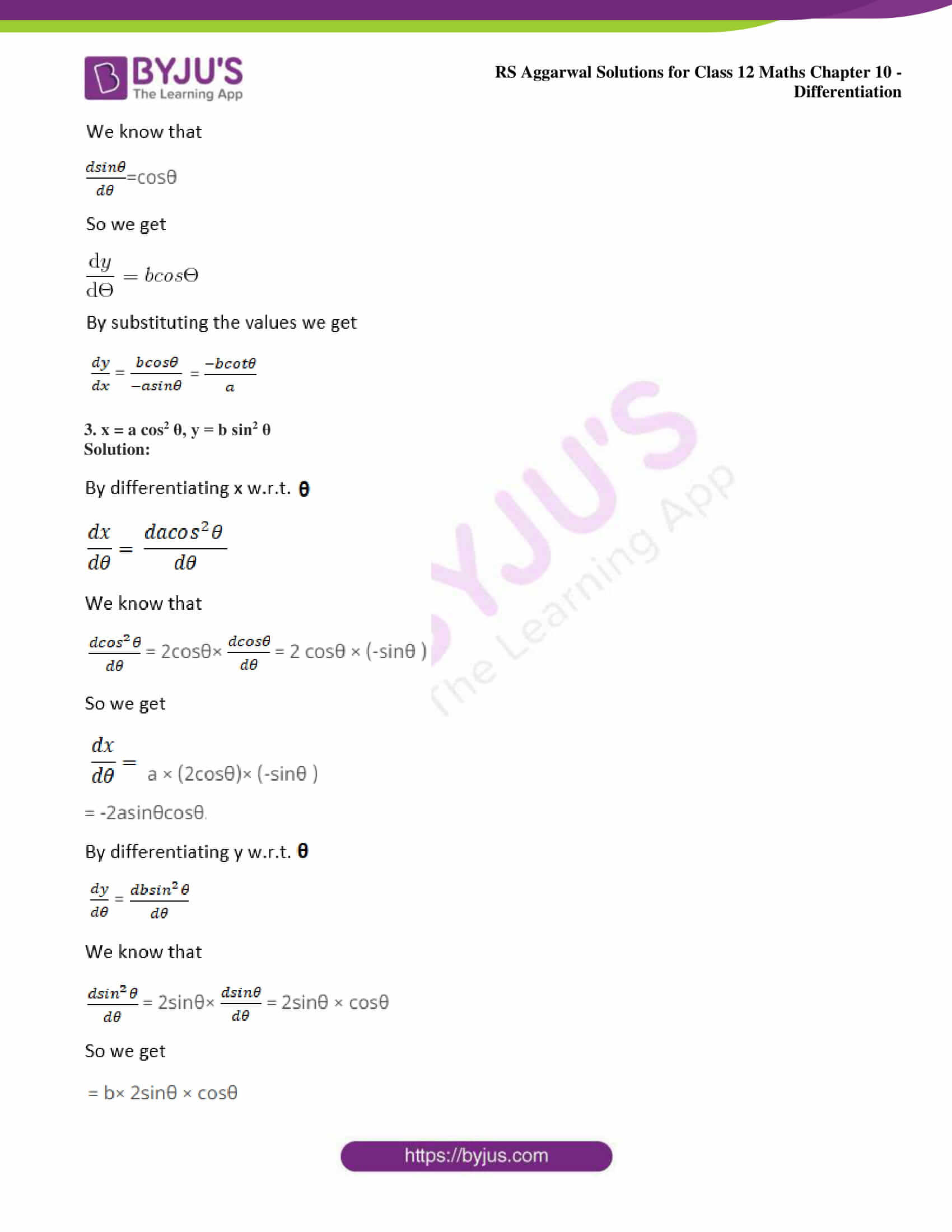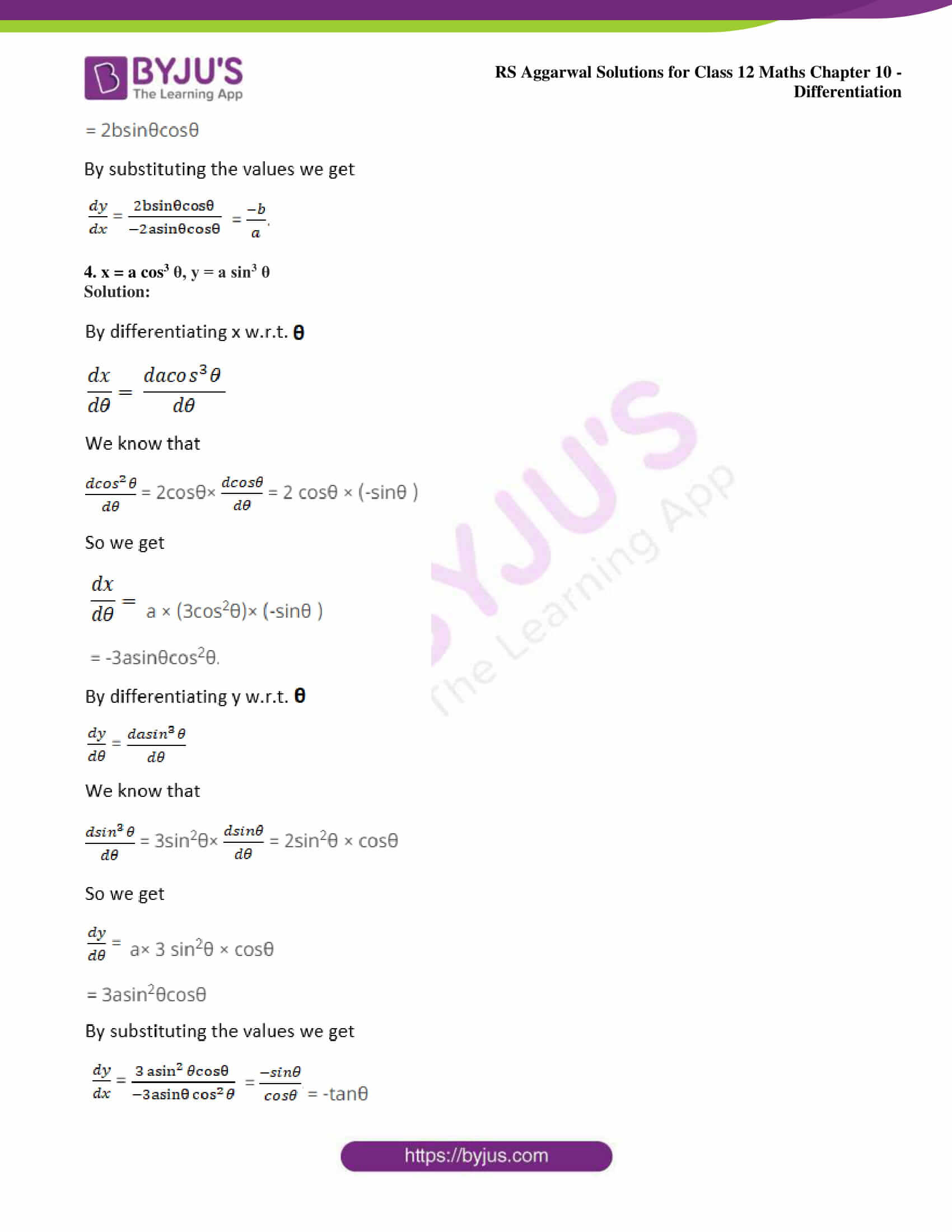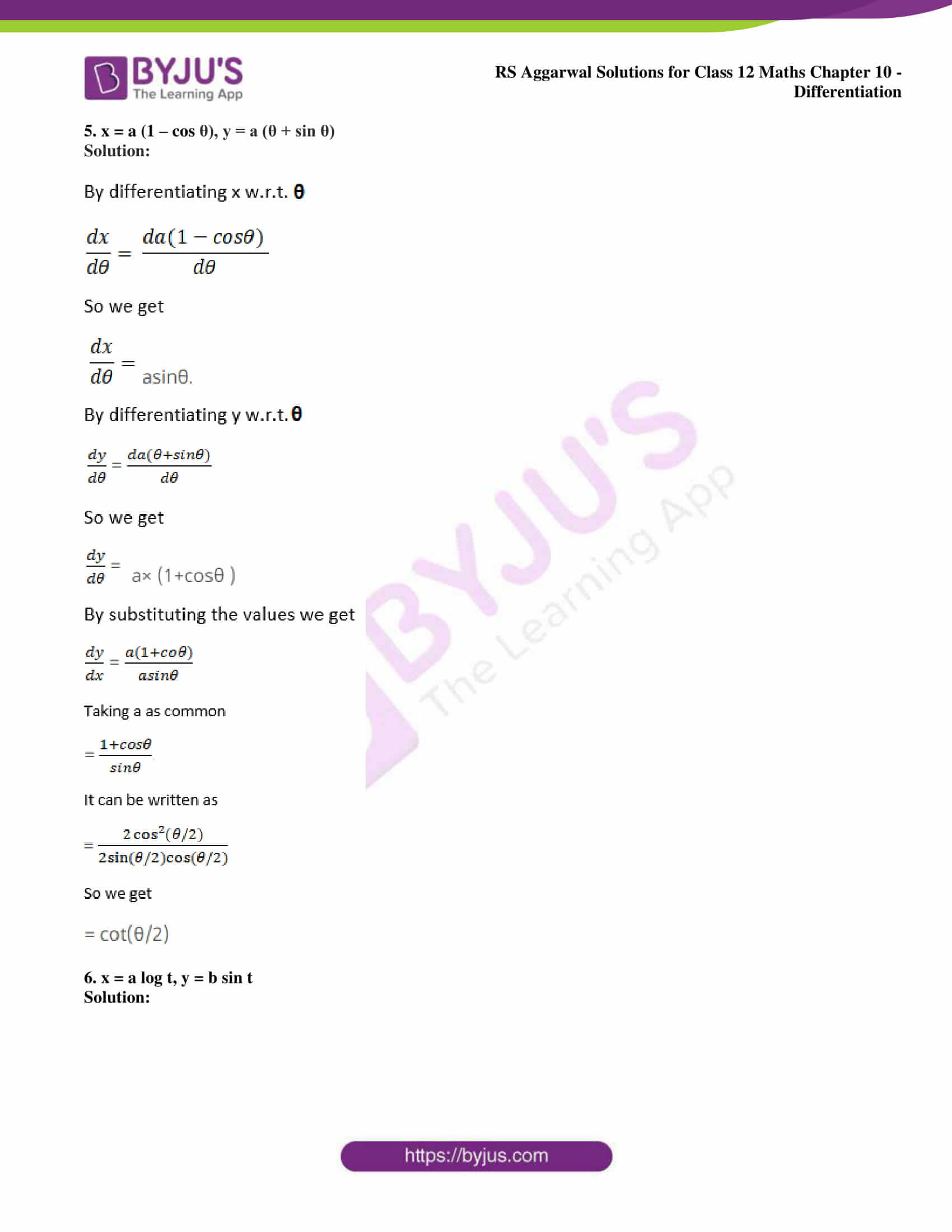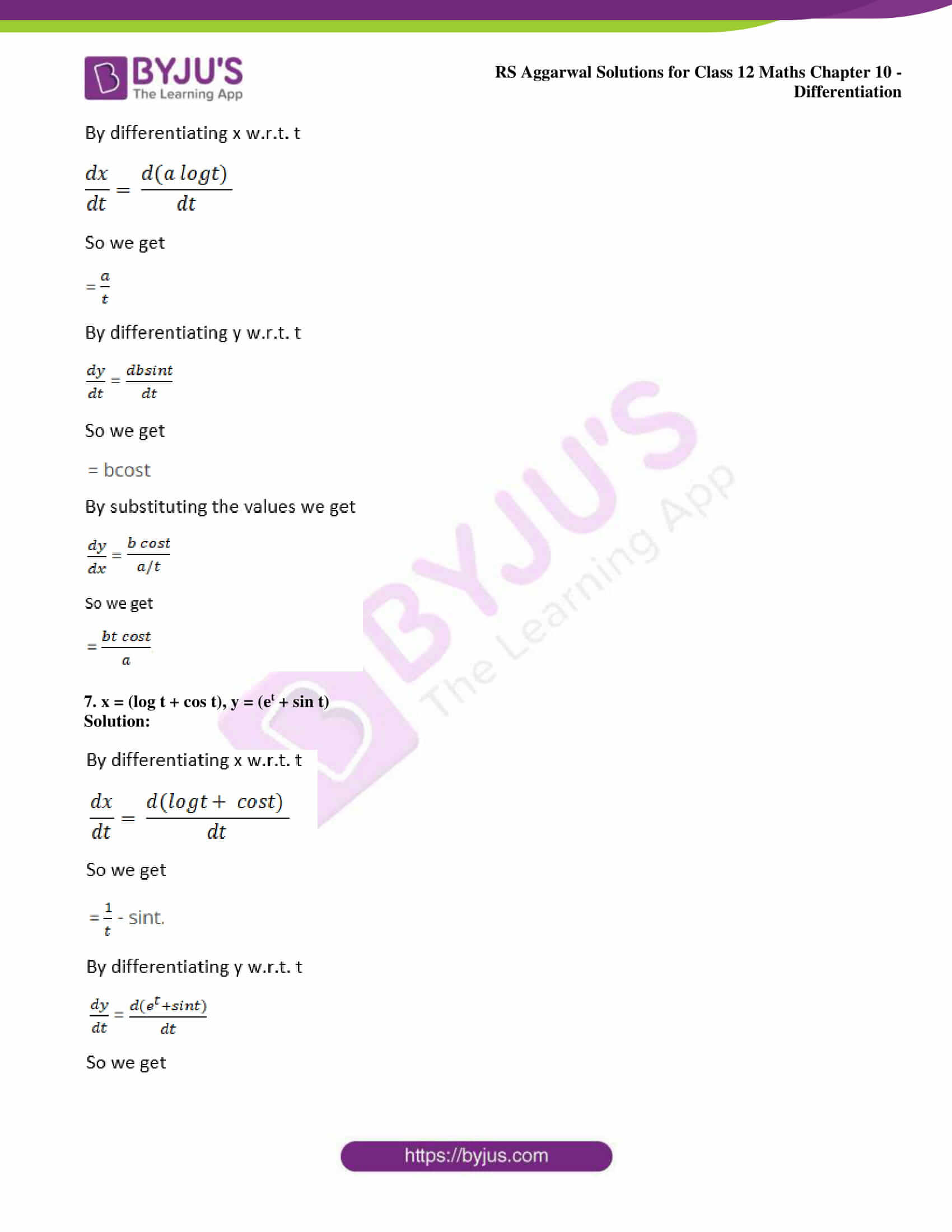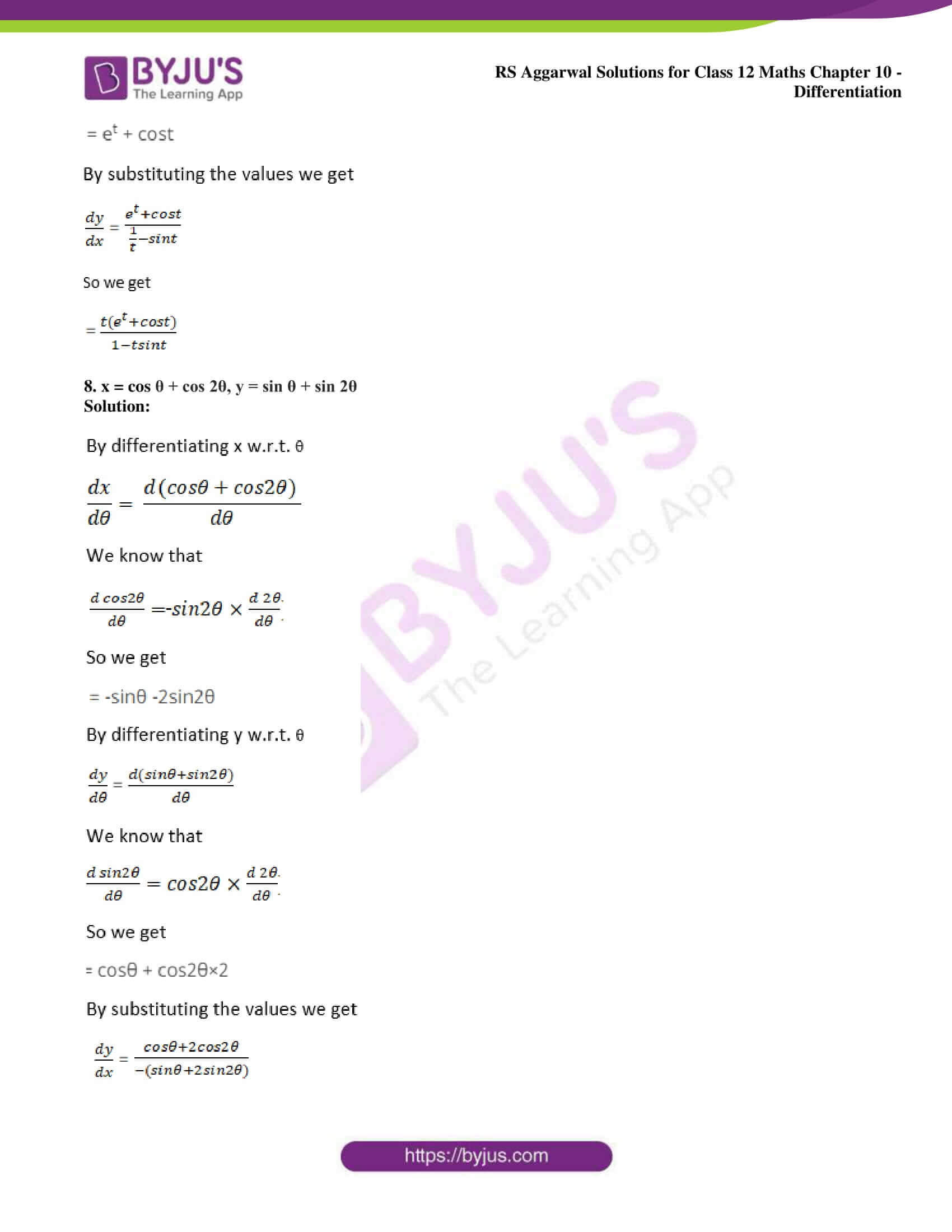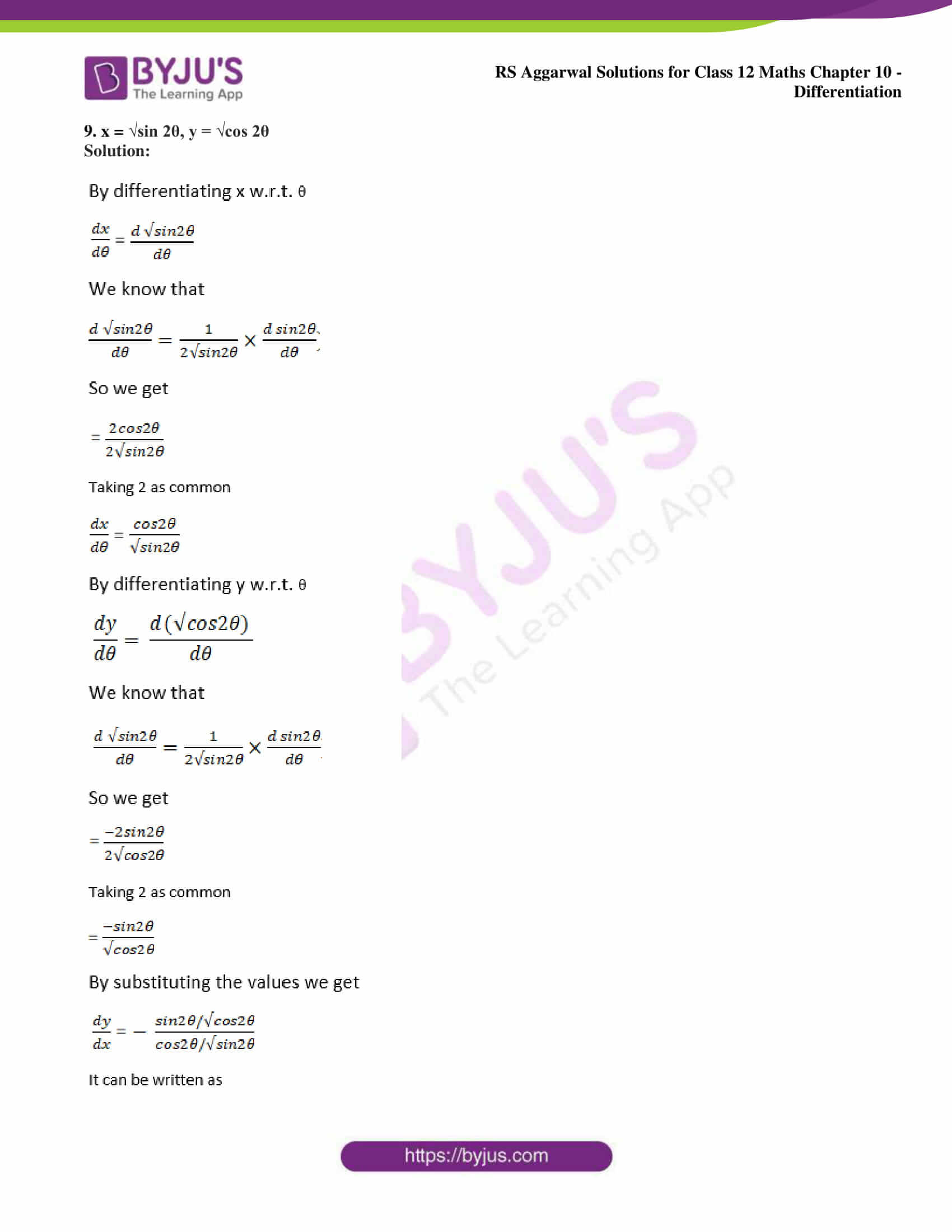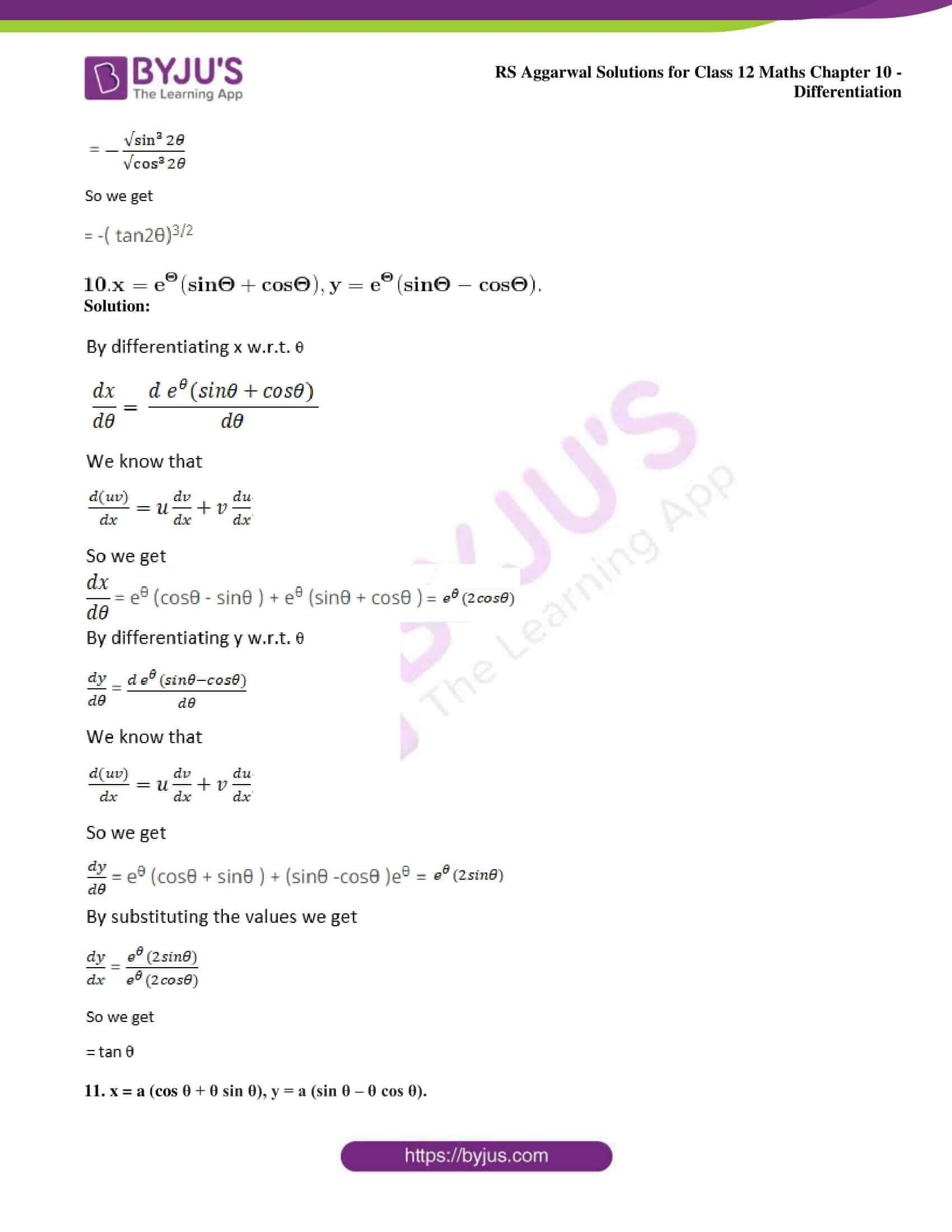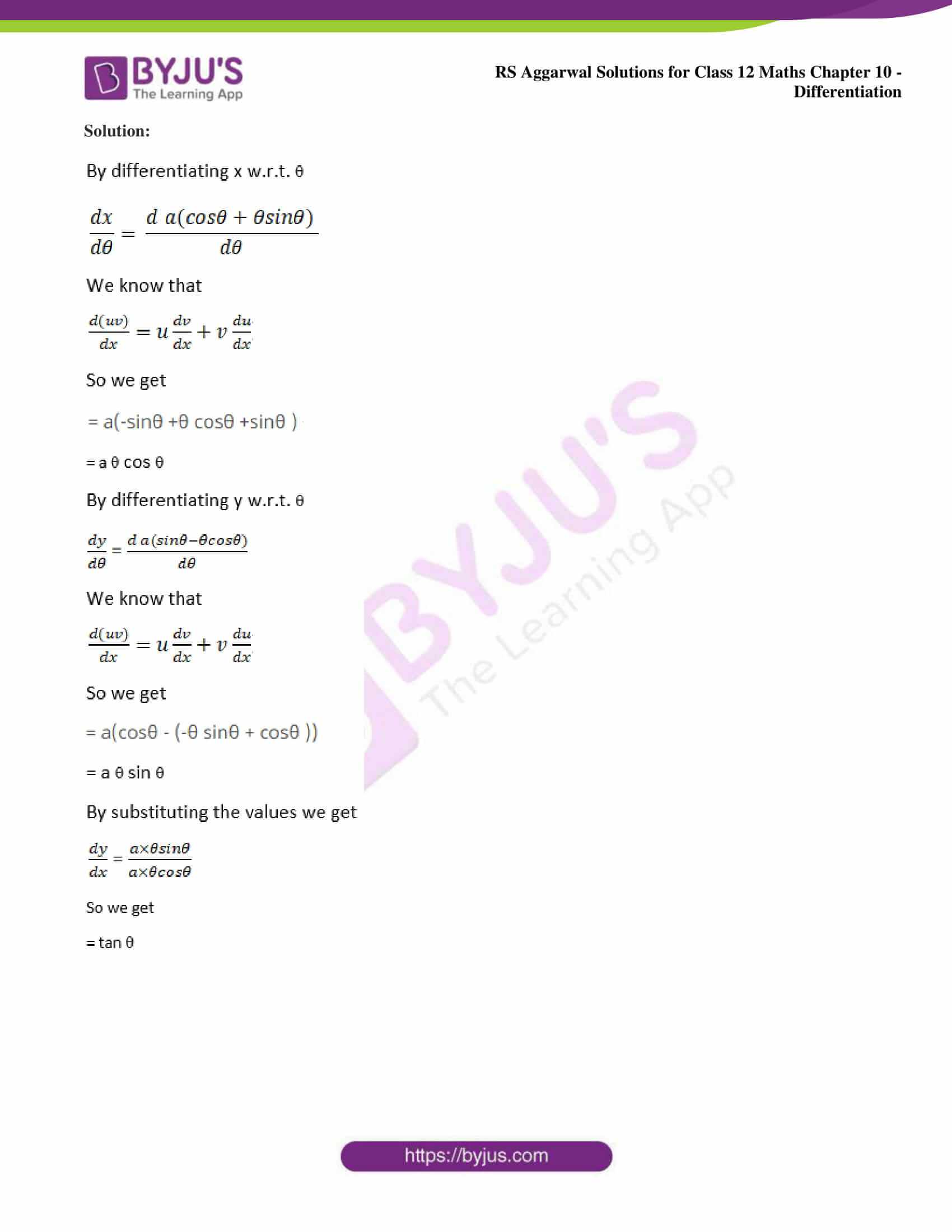### Access other exercise solutions of Class 12 Maths Chapter 10: Differentiation

Exercise 10A Solutions

Exercise 10B Solutions

Exercise 10C Solutions

Exercise 10D Solutions

Exercise 10E Solutions

Exercise 10F Solutions

Exercise 10G Solutions

Exercise 10H Solutions

Exercise 10J Solutions

### Access RS Aggarwal Solutions for Class 12 Maths Chapter 10: Differentiation Exercise 10I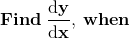1. x = at2, y = 2at

Solution: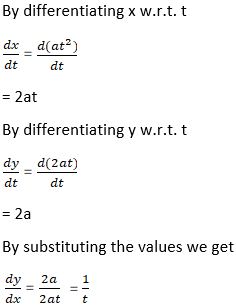2. x = a cos θ, y = b sin θ

Solution: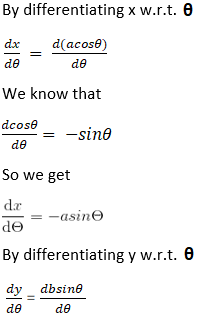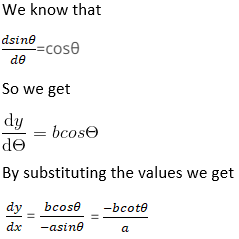3. x = a cos2 θ, y = b sin2 θ

Solution: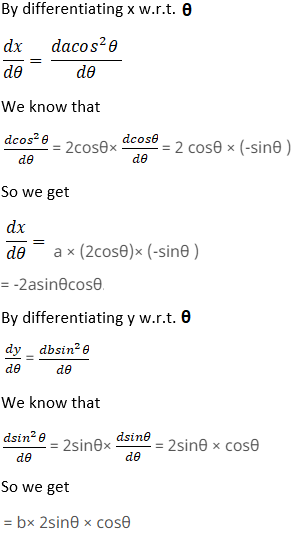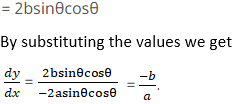4. x = a cos3 θ, y = a sin3 θ

Solution: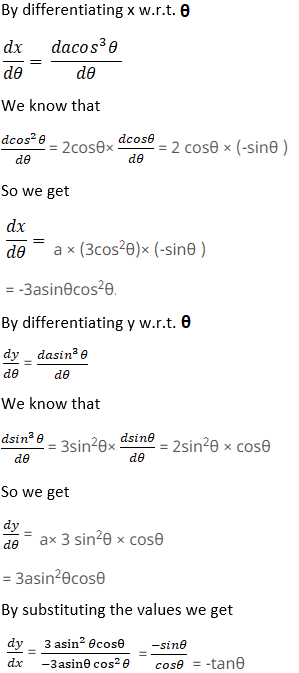5. x = a (1 – cos θ), y = a (θ + sin θ)

Solution: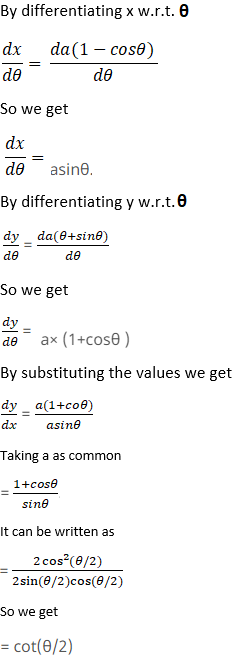6. x = a log t, y = b sin t

Solution: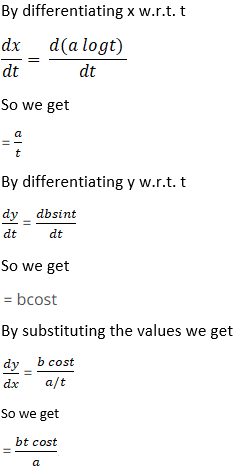7. x = (log t + cos t), y = (et + sin t)

Solution: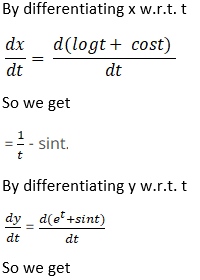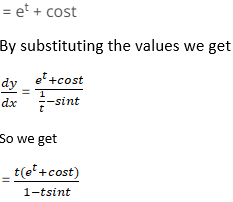8. x = cos θ + cos 2θ, y = sin θ + sin 2θ

Solution: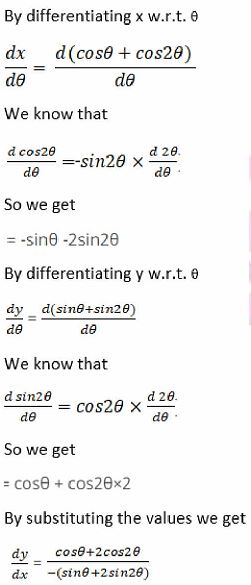9. x = √sin 2θ, y = √cos 2θ

Solution: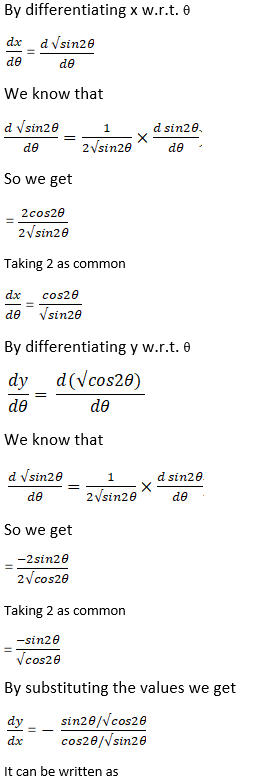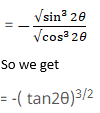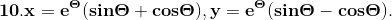Solution: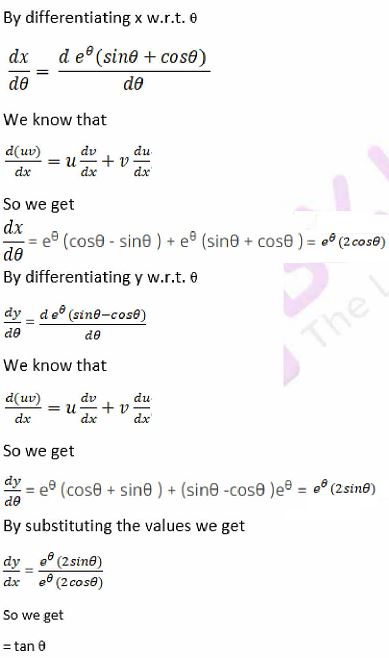11. x = a (cos θ + θ sin θ), y = a (sin θ – θ cos θ).

Solution: